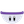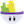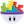#### Howdy, Stranger!

It looks like you're new here. If you want to get involved, click one of these buttons!

# Position of rotation

Posts: 23MemberThe default rotation of an actor is in the middle of it. Is it possible to change where it rotates for example the middle of the left side of a rectangle. Thanks

• Posts: 450MemberPlenty of examples in this thread.

Found by searching in the forums• Posts: 2,824Member, Sous Chef, PROedited January 2016

There is a new (much easier) way now. Just use Center of Mass.

COM.png 40.7K
• Posts: 23Member@RThurman said:
There is a new (much easier) way now. Just use Center of Mass.

Thank you for answering. But when I change the center of mass the whole actor orbits the point like earth around the sun. I need it like a clock hand rotating on its right endpoint (landscape) on a clock. Is this possible? thanks

• Posts: 2,824Member, Sous Chef, PROYes -- it can rotate like a clock hand.
Perhaps you put in the wrong coordinates?
The 0,0 point is the center of the actor. So if your clock hand is 100px long and 10px high, the X,Y coordinates for the left end would be -50,0 .

• Posts: 23Member@RThurman said:
Yes -- it can rotate like a clock hand.
Perhaps you put in the wrong coordinates?
The 0,0 point is the center of the actor. So if your clock hand is 100px long and 10px high, the X,Y coordinates for the left end would be -50,0 .

Thank you again I did have the cords wrong and it worked with the clock hand that is rotating normaly but I also have another clock hand that has this programming

Constrain: self.rotation to: (111sin( *44))+(66cos( 27))+(221sin( 33))+(111cos( 34)+66)+(33cos( 211)-22)+(112sin( *33)+180)

Which makes the clock hand rotate randomly in both clockwise and counter clock wise motions. And I made clock hand have he same dimensions as the other hand (100,10) and the same center of mass (-50,0) but it still rotates from the middle. Is there any way to make this work like the other clock hand but with the random rotations? thank you for all your help.

• London, UK.Posts: 12,822Memberedited January 2016

@virinder01 said:
Constrain: self.rotation to: (111sin( *44))+(66cos( 27))+(221sin( 33))+(111cos( 34)+66)+(33cos( 211)-22)+(112sin( *33)+180)

EDIT [RThurman's correction below includes the missing self.times]

In your other clock actor (the one without the random bidirectional rotation), how are you rotating the clock hand (by that I mean, which behaviour are you using) ?

• Posts: 2,824Member, Sous Chef, PROHmm..... it seems that the constrain behavior does not (yet) play fair with the new center of mass system.

I would suggest that you use a rotate behavior and put your formula into the "speed". To get both clockwise and counterclockwise you will need to modify the formula by removing the last "+180" from the equation. Would look like this:
`(111*sin(self.Time*44))+(66*cos(self.Time*27))+(221*sin(self.Time*33))+(111*cos(self.Time*36)+66)+(33*cos(self.Time*221)-22)+(112*sin(self.Time *33))`

• Posts: 23Member@Socks said:

@virinder01 said:
Constrain: self.rotation to: (111sin( *44))+(66cos( 27))+(221sin( 33))+(111cos( 34)+66)+(33cos( 211)-22)+(112sin( *33)+180)

Your code is messed up, the forum treats asterisks as style tags - you need to insert a space after each asterix or use the 'code' tag.

`Constrain: self.rotation to: (111*sin( *44))+(66*cos( *27))+(221*sin( *33))+(111*cos( *34)+66)+(33*cos( *211)-22)+(112*sin( *33)+180)`

In your other clock actor (the one without the random bidirectional rotation), how are you rotating the clock hand (by that I mean, which behaviour are you using) ?

In my first clock hand it is just a timer rotating the clock hand clockwise at 150 speed every 0.01 seconds.

@RThurman said:
Hmm..... it seems that the constrain behavior does not (yet) play fair with the new center of mass system.

I would suggest that you use a rotate behavior and put your formula into the "speed". To get both clockwise and counterclockwise you will need to modify the formula by removing the last "+180" from the equation. Would look like this:
`(111*sin(self.Time*44))+(66*cos(self.Time*27))+(221*sin(self.Time*33))+(111*cos(self.Time*36)+66)+(33*cos(self.Time*221)-22)+(112*sin(self.Time *33))`

When I put the formula back into the speed it just rotates at a speed slower than 150.

• Posts: 2,824Member, Sous Chef, PROHere is an example:

• London, UK.Posts: 12,822Memberedited January 2016

@virinder01 said:
In my first clock hand it is just a timer rotating the clock hand clockwise at 150 speed every 0.01 seconds.

Are you able to say how are you rotating the clock hand (by that I mean, which behaviour are you using) . . . ?

• London, UK.Posts: 12,822Memberedited January 2016

@RThurman said:
Hmm..... it seems that the constrain behavior does not (yet) play fair with the new center of mass system.

I would suggest that you use a rotate behavior and put your formula into the "speed". To get both clockwise and counterclockwise you will need to modify the formula by removing the last "+180" from the equation. Would look like this:
`(111*sin(self.Time*44))+(66*cos(self.Time*27))+(221*sin(self.Time*33))+(111*cos(self.Time*36)+66)+(33*cos(self.Time*221)-22)+(112*sin(self.Time *33))`

By the way there is a fair bit of duplication in his equation, you could reduce it a little, like this:

33 *cos(self.Time *221)
+66 *cos(self.Time *27)
+111 *cos(self.Time *36)
+111 *sin(self.Time *44)
+333 *sin(self.Time *33)
+224

• Posts: 23MemberThank you so much! It worked!

• Posts: 2,824Member, Sous Chef, PRO@virinder01 said:
Thank you so much! It worked!

You are welcome. Glad its working.

@Socks said:
By the way there is a fair bit of duplication in his equation, you could reduce it a little, like this:

33 *cos(self.Time *221)
+66 *cos(self.Time *27)
+111 *cos(self.Time *36)
+111 *sin(self.Time *44)
+333 *sin(self.Time *33)
+224

Trig Magic! You Wizard!

• London, UK.Posts: 12,822Memberedited January 2016

@RThurman said:

@virinder01 said:
Thank you so much! It worked!

You are welcome. Glad its working.

@Socks said:
By the way there is a fair bit of duplication in his equation, you could reduce it a little, like this:

33 *cos(self.Time *221)
+66 *cos(self.Time *27)
+111 *cos(self.Time *36)
+111 *sin(self.Time *44)
+333 *sin(self.Time *33)
+224

Trig Magic! You Wizard!

I just added up the angles that were progressing at the same speed and totalled all the +X and -X stuff, just a bit of equation housekeeping stuff. By the way I'm not sure the idea of removing the last "+180" from the equation (when used as the speed for Rotation to ensure CCW and CW movement) makes sense as where it is in the formula means it's just an offset, it just means the starting angle is different, there will still be the same amount of CW and CWW movement (unless I misunderstood and you are seeing a fixed starting angle). Anyhow nice and straightforward solution regardless, reminds me of your orbiting actor thinking, taking a bunch of constrains and trigonometry and replacing them all with a simple move+rotate !

• Posts: 2,824Member, Sous Chef, PRO@Socks said:
Anyhow nice and straightforward solution regardless, reminds me of your orbiting actor thinking, taking a bunch of constrains and trigonometry and replacing them all with a simple move+rotate !

It is best to avoid using the constrain behavior on any actor attribute that is involved with physics. It seems to me that constrains should only be used for attributes which only secondarily play into physics attributes. (But I've certainly broken that general rule many times in the past.)